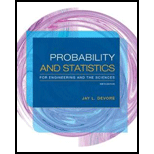# a. Show that if X has a normal distribution with parameters µ and σ , then Y = aX + b (a linear function of X ) also has a normal distribution. What are the parameters of the distribution of Y [i.e., E ( Y ) and V ( Y )]? [ Hint : Write the cdf of Y , P ( Y ≤ y ), as an integral involving the pdf of X , and then differentiate with respect to y to get the pdf of Y .] b. If, when measured in °C, temperature is normally distributed with mean 115 and standard deviation 2, what can be said about the distribution of temperature measured in °F?### Probability and Statistics for Eng...

9th Edition
Jay L. Devore
Publisher: Cengage Learning
ISBN: 9781305251809### Probability and Statistics for Eng...

9th Edition
Jay L. Devore
Publisher: Cengage Learning
ISBN: 9781305251809

#### Solutions

Chapter
Section
Chapter 4.3, Problem 57E
Textbook Problem

## a. Show that if X has a normal distribution with parameters µ and σ, then Y = aX + b (a linear function of X) also has a normal distribution. What are the parameters of the distribution of Y [i.e., E(Y) and V(Y)]? [Hint: Write the cdf of Y, P(Y ≤ y), as an integral involving the pdf of X, and then differentiate with respect to y to get the pdf of Y.]b. If, when measured in °C, temperature is normally distributed with mean 115 and standard deviation 2, what can be said about the distribution of temperature measured in °F?

Expert Solution

a.

To determine

Prove that Y has a normal distribution.

Find the parameters of Y.

### Explanation of Solution

Given info:

The random variable X has a normal distribution with parameters μ and σ. A linear function of X is Y, such that Y=aX+b.

Calculation:

The cumulative distribution function (cdf) of the random variable Y at y is:

FY(y)=P(Yy)=P(aX+by)=P(aXyb)=P(Xyba)

=FX(yba)=Φ(yba), (X has a normal distribution).

The probability density function (pdf) of Y, fY(y) can be obtained by differentiating the cdf of Y with respect to y.

fY(y)=ddyFY(y)=ddyFX(yba)=fX(yba)(1a)=1afX(yba).

Now, pdf of the normal random variable X with parameters μ and σ is known to be:

fX(x)={12πσe(xμ)22σ2, <x<,<μ<,σ>00,                      otherwise.

Replace x=yba in the pdf of X:

fX(yba)=12πσe(ybaμ)22σ2=12πσe(ybaμa)22σ2=12πσe(ybaμ)22a2σ2

Expert Solution

b.

To determine

Describe the distribution of the temperature measured in °F, if it is known that the temperature measured in °C has a normal distribution with mean 115 and standard deviation 2.

### Want to see the full answer?

Check out a sample textbook solution.See solution

### Want to see this answer and more?

Bartleby provides explanations to thousands of textbook problems written by our experts, many with advanced degrees!

See solution